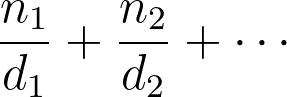# Adding Fractions Calculator

Created by Wojciech Sas, PhD candidate
Reviewed by Bogna Szyk and Jack Bowater
Last updated: Nov 29, 2021

This adding fractions calculator helps you evaluate the sum of up to five fractions in the blink of an eye. If you're looking to convert a fraction to a percent, this calculator can help. In the article below, you'll not only find how to add fractions, but also how to deal with subtraction. Are you struggling with adding fractions with unlike denominators? Read on to never have that problem again! After you're done here, the addition of fractions will never be a horror again!

Check out our other useful tools:

## How to add fractions?

A fraction is a number formed from the ratio of two numbers (A and B). These numbers are typically integers (whole numbers), so that:

fraction = A/B.

It turns out you can convert any decimal to a fraction. As a result, you can add as many decimals as you like together by treating them as if they were fractions. Whenever we want to add two fractions with a common denominator, let's say 2/7 and 3/7, we need to add numerators, while the denominator remains the same:

2/7 + 3/7 = (2+3)/7 = 5/7.

But how to add fractions with different denominators?

## Adding fractions with unlike denominators

When the numbers have different denominators, the addition of fractions is a bit more challenging, as you can't just add the numerators like before. The trick is to use common denominators. Let's see how it works. Let's say we want to add 1/2 and 1/3:

• 1/2 + 1/3 = …
• Find the common denominator. To do so, we can estimate the least common multiple (LCM) of 2 and 3. LCM(2,3) = 6
• Expand each fraction so that the denominator is this LCM, in this case 6: 1/2 = 3/6, 1/3 = 2/6
• As we know how to add fractions with the same denominator, you can just add these fractions normally: … = 3/6 + 2/6 = 5/6

There are also other equivalent fractions to this result, such as 10/12, 15/18, to name a few. However, it's convenient to present the result in its simplest form.

## Adding and subtracting fractions

Now we know how the addition of fractions works, even when adding fractions with unlike denominators - cool! But what about subtraction? Is it that simple too?

You can use this adding fractions calculator for subtracting fractions as well. We just need to remember that the subtraction of a fraction is just like addition. For example, what is 3/9 - 2/8?

• Change the subtraction to an addition: 3/9 - 2/8 = 3/9 + (-2/8)
• To make your life easier, simplify the fractions as much as possible. Find the greatest common factor for each pair of numerators and denominators: GCF(3,9) = 3, GCF(2,8) = 2
• Rewrite the expression as: 1/3 + (-1/4)
• The rest is the same as standard addition:
• Work out the common denominator: LCM(3,4) = 12
• Expand the fractions and add them: 4/12 + (-3/12) = 1/12
• Which is the same as: 1/3 - 1/4 = 1/12

Additionally, in our calculator there is no difference between -1/4 or 1/-4 - our adding fractions calculator will treat these expressions the same!

## Addition of fractions in practice - how to use adding fractions calculator?

Imagine a story - you are at a party with some of your friends. And there it comes - the hunger! What's even worse, you've just realized there is nothing left in the fridge.

A solution is simple - you are going to order a pizza, or two, or even more. You have to decide! The point is your favorite pizzeria sells pizzas as a whole, but you have an innovative method that can cut pizzas into 6, 8, or 12 slices. Everyone wants a certain fraction of a pizza: five of you want 4 of 6-slice pizzas, four of you prefer 3 of the 8-slice pizzas, and the remaining three will be happy with 6 of 12-slice ones. The main question is: How many pizzas should we order?

You can always evaluate it by hand, but why don't you try our adding fractions calculator and saving your time! Input the following values:

1. 5*4 and 6 for the first number
2. 4*3 and 8 for the second number
3. 3*6 and 12 for the third one

The outcome is 19/3, or 6 1/3, as a mixed number. It means that six pizzas won't be enough, so you'd better order seven!
Moreover, you can choose the step by step solution to see all the calculations with explanations. You are all welcome to read it while enjoying your delicious meal!

Wojciech Sas, PhD candidateValues (you can enter up to 5 fractions)
They are in
simple fraction form
1st fraction
Numerator (n₁)
Denominator (d₁)
2nd fraction
Numerator (n₂)
Denominator (d₂)
Show step by step solution?
No
People also viewed…

### Cholesky decomposition

The Cholesky decomposition calculator lets you quickly and easily obtain the lower triangular matrix of the Cholesky factorization. Pick between a 2×2, 3×3, or a 4×4 matrix.

### Circle skirt

Circle skirt calculator makes sewing circle skirts a breeze.

### Plastic Footprint

Find out how much plastic you use throughout a year with this plastic footprint calculator. Rethink your habits, reduce your plastic waste, and make your life a little greener.

### Semicircle area

This semicircle area calculator determines the area of a half circle, as well as the circumference of a semicircle.